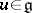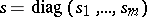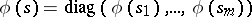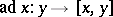# Lie algebra, algebraic

(diff) ← Older revision | Latest revision (diff) | Newer revision → (diff)
The Lie algebra of an algebraic subgroup (see Algebraic group) of the general linear group of all automorphisms of a finite-dimensional vector spaceover a field. Ifis an arbitrary subalgebra of the Lie algebra of all endomorphisms of, there is a smallest algebraic Lie algebra containing; it is called the algebraic envelope (or hull) of the Lie subalgebra. For a Lie algebraover an arbitrary algebraically closed fieldto be algebraic it is necessary that together with every linear operatorits semi-simple and nilpotent componentsandshould lie in(see Jordan decomposition). This condition determines the so-called almost-algebraic Lie algebras. However, it is not sufficient in order thatbe an algebraic Lie algebra. In the case of a fieldof characteristic 0, a necessary and sufficient condition for a Lie algebrato be algebraic is that, together withand, all operators of the formshould lie in, whereis an arbitrary-linear mapping frominto. The structure of an algebraic algebra has been investigated
in the case of a field of characteristic.
A Lie algebraover a commutative ringin which for any elementthe adjoint transformationdefined onis the root of some polynomial with leading coefficient 1 and remaining coefficients from. A finite-dimensional Lie algebra over a fieldis an algebraic Lie algebra. The converse is false: Over any fieldthere are infinite-dimensional algebraic Lie algebras with finitely many generators . A number of questions about algebraic Lie algebras have been solved in the class of nil Lie algebras (cf. Lie algebra, nil).